# Double-Box Branchlines

New for February 2006! We took a closer look at the double-box branchline coupler, a.k.a. the three-section hybrid according to Pozar's Microwave Engineering book (order it here!) There is precious little in the literature about this, but you can trace it back to the 1950s when it was described in a MTT paper.

We had seen more than one solution to the impedances, the picture in Pozar's book clearly show fifty-ohms as the predominant impedance Z1. But we had also seen 35.35 ohms. What gives?

We tried varying the three impedances to get different values, using Agilent's ADS. We looked for solutions that gave equal split (-3 dB), infinite return loss and isolation of the fourth port. It turns out there is more than one way to skin this cat! We found that the "end" impedances Z2 of the box must remain at [1+sqrt(2)] x Z0 (120.8 ohms in a fifty-ohm system), but Z1 and Z3 can be varied according to:

Z3=sqrt(2)x(Z1^2)/Z0

Thanks for the correction, Steve!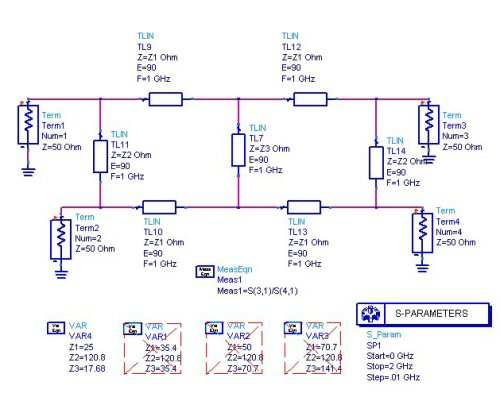Here we will plot the four solutions we came up with to compare their frequency responses. This one has Z1=25 ohms.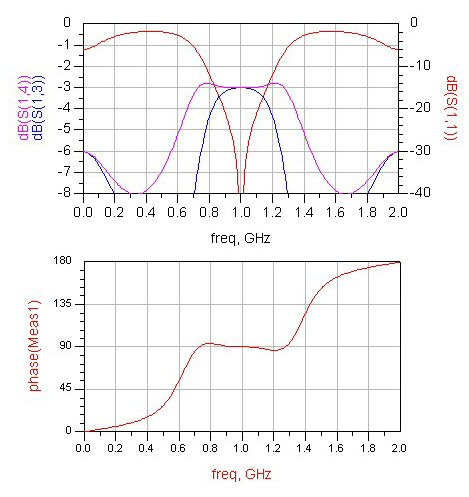This one has Z1=35.35 ohms: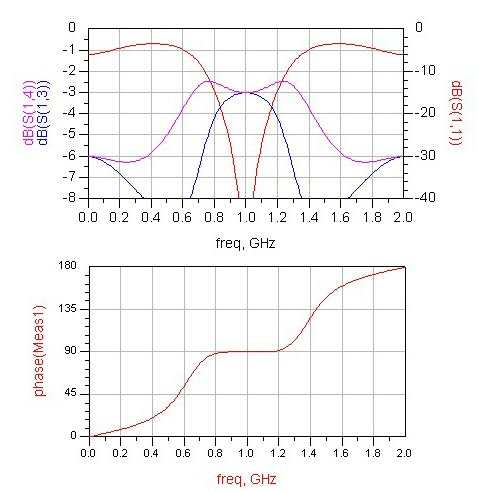This one has Z1=50 ohms: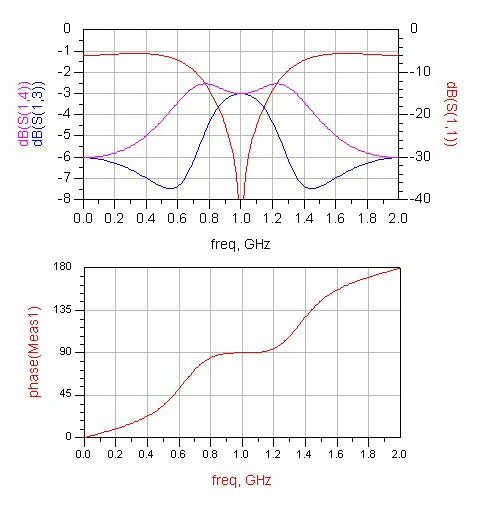This one has Z1=70.7 ohms: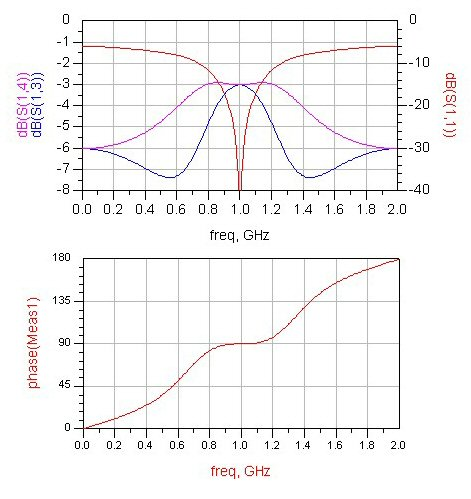Now that's something you won't learn anywhere else but Microwaves101.com!

Author : Unknown Editor